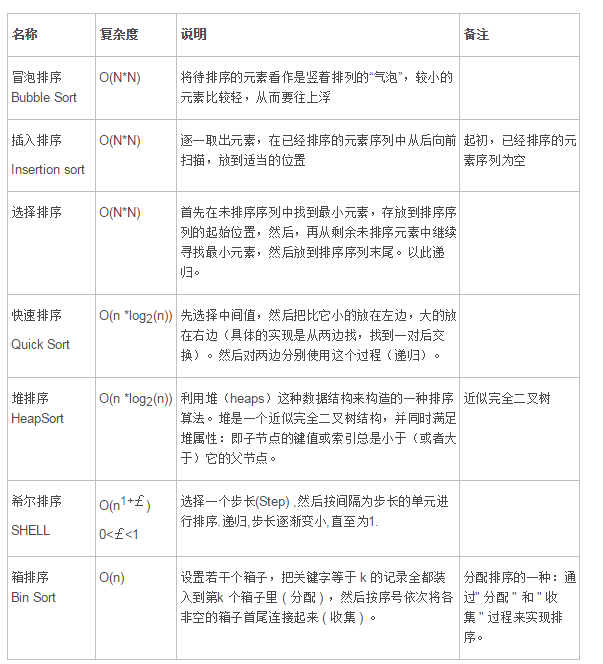## 算法_bingoabin的博客-程序员秘密

### 1.算法定义

①有穷性（Finiteness）：算法的有穷性是指算法必须能在执行有限个步骤之后终止；
②确切性(Definiteness)：算法的每一步骤必须有确切的定义；
③输入项(Input)：一个算法有0个或多个输入，以刻画运算对象的初始情况，所谓0个输 入是指算法本身定出了初始条件；
④输出项(Output)：一个算法有一个或多个输出，以反映对输入数据加工后的结果。没 有输出的算法是毫无意义的；
⑤可行性(Effectiveness)：算法中执行的任何计算步骤都是可以被分解为基本的可执行 的操作步，即每个计算步都可以在有限时间内完成（也称之为有效性）；
⑥高效性(High efficiency)：执行速度快，占用资源少；
⑦健壮性(Robustness)：对数据响应正确。

### 3.时间复杂度计算方法

1.一个算法执行所耗费的时间，从理论上是不能算出来的，必须上机运行测试才能知道。但我们不可能也没有必要对每个算法都上机测试，只需知道哪个算法花费的时间多，哪个算法花费的时间少就可以了。并且一个算法花费的时间与算法中语句的执行次数成正比例，哪个算法中语句执行次数多，它花费时间就多。

2.一般情况下，算法的基本操作重复执行的次数是模块n的某一个函数f（n），因此，算法的时间复杂度记做：T（n）=O（f（n））。随着模块n的增大，算法执行的时间的增长率和f（n）的增长率成正比，所以f（n）越小，算法的时间复杂度越低，算法的效率越高。

3.常见的时间复杂度

1.O(n)，O(n^2)， 立方阶O(n^3),…， k次方阶O(n^k) 为多项式阶时间复杂度，分别称为一阶时间复杂度，二阶时间复杂度。。。。
2.O(2^n)，指数阶时间复杂度，该种不实用
3.对数阶O(log2n), 线性对数阶O(nlog2n)，除了常数阶以外，该种效率最高
``````例：算法：
for（i=1;i<=n;++i）
{
for(j=1;j<=n;++j)
{
c[ i ][ j ]=0; //该步骤属于基本操作 执行次数：n^2
for(k=1;k<=n;++k)
c[ i ][ j ]+=a[ i ][ k ]*b[ k ][ j ]; //该步骤属于基本操作 执行次数：n^3
}
}
则有 T（n）= n^2+n^3，根据上面括号里的同数量级，我们可以确定 n^3为T（n）的同数量级
则有f（n）= n^3，然后根据T（n）/f（n）求极限可得到常数c
则该算法的 时间复杂度：T（n）=O（n^3)
``````

### 4.讨论

“大O记法”：在这种描述中使用的基本参数是 n，即问题实例的规模，把复杂性或运行时间表达为n的函数。这里的“O”表示量级 (order)，比如说“二分检索是 O(logn)的”,也就是说它需要“通过logn量级的步骤去检索一个规模为n的数组”记法 O ( f(n) )表示当 n增大时，运行时间至多将以正比于 f(n)的速度增长。

``````O(1)

Temp=i;i=j;j=temp;

O(n^2)

2.1. 交换i和j的内容
sum=0；                 （一次）
for(i=1;i<=n;i++)       （n次 ）
for(j=1;j<=n;j++) （n^2次 ）
sum++；       （n^2次 ）

2.2.
for (i=1;i<n;i++)
{
y=y+1;         ①
for (j=0;j<=(2*n);j++)
x++;        ②
}

语句2的频度是(n-1)*(2n+1)=2n^2-n-1
f(n)=2n^2-n-1+(n-1)=2n^2-2
该程序的时间复杂度T(n)=O(n^2).

O(n)

2.3.
a=0;
b=1;                      ①
for (i=1;i<=n;i++) ②
{
s=a+b;　　　　③
b=a;　　　　　④
a=s;　　　　　⑤
}

语句2的频度： n,
语句3的频度： n-1,
语句4的频度：n-1,
语句5的频度：n-1,
T(n)=2+n+3(n-1)=4n-1=O(n).

O(log2n )

2.4.
i=1;       ①
while (i<=n)
i=i*2; ②

设语句2的频度是f(n),   则：2^f(n)<=n;f(n)<=log2n
取最大值f(n)= log2n,
T(n)=O(log2n )

O(n^3)

2.5.
for(i=0;i<n;i++)
{
for(j=0;j<i;j++)
{
for(k=0;k<j;k++)
x=x+2;
}
}

``````

### 5.常用排序### 将java对象存储到redis数据库（使用序列化和反序列化）_二十亩鱼的博客-程序员秘密

Redis不支持直接将Java对象存储到数据库中，所以需要将java对象进行序列化得到字节数组，然后将字节数组存入到redis中，需要数据的时候就从redis数据库中取出字节数组，再经过反序列化将自己数组转换成对象使用（jdk序列化性能比谷歌公司的Protobuf序列化性能要差一些，而且序列化后的字节长度要也会长一些，所以推荐使用Protobuf，Protobuf如何进行序列化请看我的另一篇帖子）

### 通过shell和redis来实现集群业务中日志的实时收集分析_weixin_33811961的博客-程序员秘密

在统计项目中，最难实施的就是日志数据的收集。日志分布在全国各个机房，而且数据量比较大，像rsync+inotify这种方式显然不能满足快速日志同步的要求。 当然大家也可以用fluentd和flume采集日志数据，除了这个我们也可以自己写一套简单的。 我写的这个日志分析系统 流程是：1   在客户端收集数据，然后通过redis pub方式把数据发给服务端  2   服务器端是r...

### Python源代码(改进版)---模板类型表的增删改查_奋斗吧，青年！的博客-程序员秘密

import pymysql## 连接数据库def connection(): connect = pymysql.Connect( host='10.203.39.62', port=3306, user='root', passwd='bdpptyfbyw', db='bdp', charset='utf8' ) return connect## 增加def in.

### libcurl linux 静态链接库_libcurl源码编译及使用_轮王寺宫的博客-程序员秘密

http://blog.csdn.net/b_h_l/article/details/199850612014.021:linux下源码编译./configure --prefix=/usr/local/curl --disable-shared --enable-static --without-libidn--without-ssl --without-librtmp --without-gn...

### Python3 writerow函数TypeError: a bytes-like object is required, not 'str'_writerow a bytes-like object is required str_779醒的博客-程序员秘密

with open(path, 'wb') as csv_file: csv_write = csv.writer(csv_file) csv_head = ["A", "B"] csv_write.writerow(csv_head)运行错误提示：TypeError: a bytes-like object is required, ...

### log_888log_Vevina888的博客-程序员秘密

# LOG ## Log简介 logging模块是Python内置的标准模块，主要用于输出运行日志，可以设置输出日志的等级、日志保存路径、日志文件回滚等；相比print，具备如下优点： 通过log的分析，可以方便用户了解系统或软件、应用的运行情况；如果你的应用log足够丰富，也可以分析以往用户的操作行为、类型喜好、地域分布或其他更多信息；如果一个应用的log同时也分了多个级...

C++面试题C++基本知识点

### c语言随机产生100个字母,C语言实现生成1到100随机数的方法_相机拿反了的博客-程序员秘密

C语言实现生成1到100随机数的方法发布时间：2020-06-29 14:08:59来源：亿速云阅读：7054作者：Leah本篇文章为大家展示了C语言实现生成1到100随机数的方法，代码简明扼要并且容易理解，绝对能使你眼前一亮，通过这篇文章的详细介绍希望你能有所收获。生成10个1～100的随机数废话不多说直接上程序。#include#include#includeintmain(){intr...